DGtal  1.4.beta
Cellular grid space and topology, unoriented and oriented cells, incidence
Author(s) of this documentation:
by Jacques-Olivier Lachaud, Bertrand Kerautret and Roland Denis

Part of the Topology package.

This part of the manual describes how to define cellular grid space or cartesian cubic spaces, as well as the main objects living in these spaces. A lot of the ideas, concepts, algorithms, documentation and code is a backport from ImaGene.

# Images and digital spaces

2D images are often seen as two dimensional arrays, where each cell is a pixel with some value (a gray level, a color). If $$\mathbf{Z}$$ is the set of integer numbers, then an image is a map from a rectangular subset of $$\mathbf{Z} \times \mathbf{Z}$$ to some space (gray levels, colors).

More generally, a nD image is a map from a parallelepipedic subset of $$\mathbf{Z}^n$$ to some space (gray levels, colors).

Many algorithms need to represent positions in images (ie pixels and voxels), in order to represent regions in images. Often, we need also to measure the shape of a region, for instance its perimeter, or we may be interested in the interface between two regions. In these cases, it is often convenient (and generally it is also the theoretic way) to represent other elements in digital spaces, such as paths in-between regions, the thin boundary or surface of a region, etc. We need in this case to represent not only the "squares" (pixels) or "cubes" (voxels) of images, but also their faces, edges, vertices.

We therefore model not only n-dimensional cells (the hypercubes in nD), but also all the lower dimensional cells of the space. For instance, in 2D, we have:

• 2-dimensional cells (closed unit square) = pixels
• 1-dimensional cells (closed unit segment) = linels
• 0-dimensional cells (closed point) = pointels

The set of all cells $$\mathbf{F}^n$$ is called the n-dimensional space of cubical complexes, or n-dimensional cellular grid space. The couple $$(\mathbf{F}^n,\subseteq)$$ is a partially ordered set or poset. Let $$X$$ be any subset of $$\mathbf{F}^n$$. The set $$\mathcal{U}=\{U \subseteq X / \forall x \in U, x^{\uparrow} \subseteq U \}$$, where $$x^{\uparrow}=\{y \in X, x \le y\}$$ or the up incident cells of x, is a topology on $$X$$, called the Aleksandrov topology. Therefore we can use standard combinatorial topology results for any subset of the cellular grid space.

Cells of the cellular grid space are illustrated below. The paving mode of digital space gives a nice illustration of what is this space. The 2-cell is in red, the 1-cell in green, the 0-cell in blue.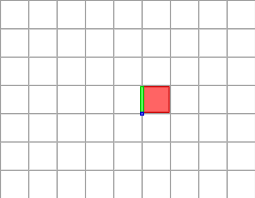Illustration of a cellular grid space with cells of different dimensions.

## Cells in the cubical grid and Khalimsky coordinates

We use now the regularity of the cubical grid to represent it efficiently. In 1D, the cubical grid is a simple line alternating closed points {k} and open unit segments (k,k+1). Khalimsky noticed that this (topological) space is homeomorphic to the integer set $$\mathbf{Z}$$, if we declare every even integer as closed and every odd integer as open.

A digital cell in 1D is thus just an integer. Its topology is defined by its parity. The cell 2k is the closed point {k}; the cell 2k+1 is the segment (k,k+1) (considered open).

In 2D, we use the fact that $$\mathbf{Z}^2$$ is a cartesian product. A digital cell in 2D is thus a couple of integer

• (2i, 2j): a pointel which is the point set {(i,j)} in the plane
• (2i+1, 2j): an horizontal linel which is the open segment {(i,i+1) x j} in the plane.
• (2i, 2j+1): a vertical linel which is the open segment {i x (j,j+1)} in the plane.
• (2i+1, 2j+1): a pixel which is the open square {(i,i+1) x (j,j+1)} in the plane.

In nD, the principle is the same. A cell is thus uniquely identifed by an n-tuple of integers whose parities define the topology of the cell. These integers are called the Khalimsky coordinates of the cell.

For instance, the pixel (x,y) of the digital space $$\mathbf{Z}^2$$ corresponds to the 2-cell (2x+1,2y+1) of the cellular grid space $$\mathbf{F}^2$$.

## Models for cellular grid spaces

Instead of chosing a specific implementation of a cellular grid space, we keep the genericity and efficiency objective of DGtal by defining a cellular grid space as the concept CCellularGridSpaceND. It provides a set of types (Cell, SCell, etc) and methods to manipulate cells of arbitrary dimension. Models of CCellularGridSpaceND are:

1. the KhalimskySpaceND template class, that allows per-dimension closure specification (open, closed or periodic).
2. the — yet to come — CodedKhalimskySpaceND class, a backport from class KnSpace of ImaGene.

The inner types are:

Methods include:

• Cell creation services
• Write accessors to cells
• Conversion signed/unsigned cells
• Cell topology services
• Direction iterator services for cells
• Unsigned cell geometry services
• Signed cell geometry services
• Neighborhood services
• Incidence services
• Interface

An comprehensive description is given in CCellularGridSpaceND.

## Creating a cellular grid space

We use hereafter the model KhalimskySpaceND. To create a 2D cellular grid space where cells have coordinates coded with standard int (32 bits generally), we can write:

#include "DGtal/topology/KhalimskySpaceND.h"
...
typedef KhalimskySpaceND< 2, int > KSpace;
Point low( -3, -4 );
Point high( 5, 3 );
// true for closed space, false for open space.
bool space_ok = K.init( low, high, true );
MyPointD Point
Definition: testClone2.cpp:383
KSpace K

Note that the cellular grid space is limited by the given bounds. Since the user has chosen a closed space, the Khalimsky coordinates of cells are bounded by:

• lower bound: (-3*2, -4*2)
• upper bound: (5*2+2, 3*2+2)

Another frequent way of constructing a cellular grid space is to start from a preexisting digital space and HyperRectDomain. You can define the associated cellular grid space as follows:

...
typedef SpaceND<3, int> Z;
typedef HyperRectDomain<Z> Domain;
...
Domain domain( a, b );
...
typedef KhalimskySpaceND< Z::dimension, Z::Integer > KSpace;
bool space_ok = K.init( domain.lowerBound(), domain.upperBound(), true );
HyperRectDomain< Space > Domain
Domain domain
HyperRectDomain< Space > Domain

If you wish to build a digital space and a HyperRectDomain from a cellular grid space K, you may write:

...
typedef SpaceND<KSpace::dimension, KSpace::Integer> Z;
typedef HyperRectDomain<Z> Domain;
Domain domain( K.lowerBound(), K.upperBound() );

Last but not least, for standard users it is generally enough to use the default types provided in DGtal/helpers/StdDefs.h.

#include "DGtal/helpers/StdDefs.h"
...
using namespace Z2i;
KSpace K; // or K2 K;
...

# Creating (unsigned) cells in a cellular grid space

There are many ways of creating cells within a cellular grid space. The simplest way is certainly to give the Khalimsky coordinates of the cell you wish to create. Its topology is then induced by its coordinates. We use generally KhalimskySpaceND::uCell for arbitrary cells, KhalimskySpaceND::uSpel for n-dimensional cells, KhalimskySpaceND::uPointel for 0-dimensional cells.

The full code of this example is in file ctopo-1.cpp. Pointels or 0-cells are displayed in blue, linels or 1-cells in green, pixels or 2-cells in red. Note that KhalimskySpaceND::uCell requires Khalimsky coordinates while the two others require digital coordinates (the topology is known in the two latter cases).

Point plow(-3,-2);
Point pup(5,3);
K.init( plow, pup, true );
Cell pixlow = K.uSpel( plow ); // pixel (-3*2+1,-2*2+1)
Cell ptlow = K.uPointel( plow ); // pointel (-3*2,-2*2)
Cell pixup = K.uSpel( pup ); // pixel (5*2+1,3*2+1)
Cell ptup1 = K.uPointel( pup );// pointel (5*2,3*2)
Cell ptup2 = K.uTranslation( ptup1, Point::diagonal() ); // pointel (6*2,4*2)
Cell linelb = K.uCell( Point( 1, 0 ) ); // linel (1,0) bottom
Cell linelt = K.uCell( Point( 1, 2 ) ); // linel (1,2) top
Cell linell = K.uCell( Point( 0, 1 ) ); // linel (0,1) left
Cell linelr = K.uCell( Point( 2, 1 ) ); // linel (2,1) right
KSpace::Cell Cell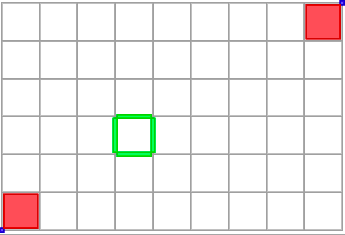Displaying some cells in different colors.

The file ctopo-1-3d.cpp shows another example in 3D:

Point plow(0,0,0);
Point pup(3,3,2);
...
Cell ptlow = K.uPointel( plow ); // pointel (0*2,0*2, 0*2)
Cell ptup1 = K.uPointel( pup ); // pointel (3*2,3*2, 2*2)
Cell ptup2 = K.uTranslation( ptup1, Point::diagonal() ); // pointel (4*2, 4*2, 3*2)
viewer << ptlow << ptup1 << ptup2;
Cell linel0 = K.uCell( Point( 1, 0, 2 ) ); // linel (2, 0, 2)
viewer << linel0; // ...
Cell surfelA = K.uCell( Point( 2, 1, 3 ) ); // surfel (2,1,3)
viewer << surfelA; //...
Cell vox1 = K.uCell( Point( 3, 3, 3 ) ); // voxel (3,3,3)
viewer << vox1;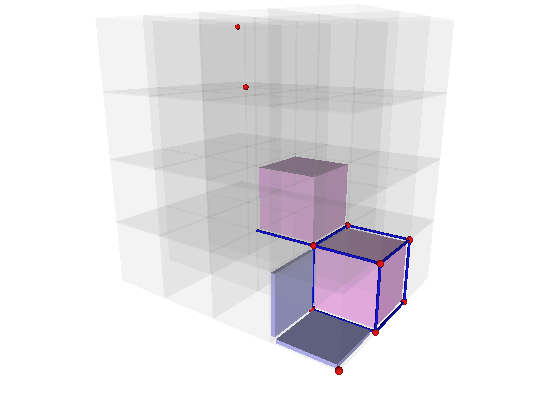Displaying some cells in different colors.

# Cells may be unsigned or signed

Up to now, we have only consider unsigned cells (type Cell). However it is often convenient to assign a sign (POS or NEG) to a cell, a kind of orientation. The sign is especially useful to define boundary operators and digital surfaces. It is also used in algebraic topological models of cubical complexes, for instance to define chains (formal sums of cells).

Signed cells have type SCell. They are created using methods KhalimskySpaceND::sCell for arbitrary cells, KhalimskySpaceND::sSpel for n-dimensional cells, KhalimskySpaceND::sPointel for 0-dimensional cells. The user gives the sign at creation, either K.POS or K.NEG if K is the space.

You may use methods KhalimskySpaceND::sSign, KhalimskySpaceND::sSetSign KhalimskySpaceND::signs, KhalimskySpaceND::unsigns, KhalimskySpaceND::sOpp, respectively to get the sign of a signed cell, to change the sign of a signed cell, to sign an unsigned cell, to unsign a signed cell, and to compute the cell with opposite sign.

All methods concerning unsigned cells are prefixed by u, all methods concerning signed cells are prefixed by s.

Note that the sign of the signed and unsigned are well taked into account in the display with Viewer3D .Visualisation of unsigned Cell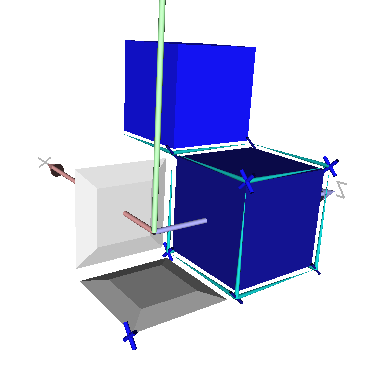Visualisation of signed Cell

# Accessing and modifying cell coordinates.

Since one does not necessarily know which model of CCellularGridSpaceND you may be using, you cannot access the cell coordinates directly. Therefore a model of CCellularGridSpaceND provides a set of methods to access and modify cell coordinates and topology. Here a few of them (with the model example KhalimskySpaceND)

# Moving within the cellular grid space

Note that you dispose also of a whole set of methods to determine cells according to different geometric queries. The following methods do not change the topology of the input cell only the coordiantes. Again the prefix u is related to method taking as input unsigned cells while the prefix s is related to signed cells:

Cell first, last; // lower and upper bounds
Cell p = first;
do
{ // ... whatever [p] is the current cell
}
while ( K.uNext( p, first, last ) );

For instance (see. khalimskySpaceScanner.cpp) you will obtain the default following scan: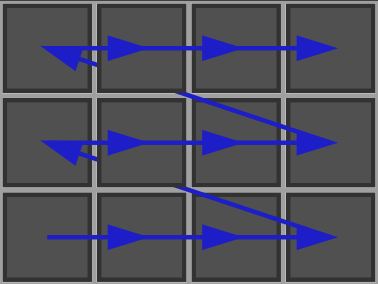Sequence of visited pixels in a scan of Khalimsky Space (standard scan order).
• The scan can also be done by explicitly controlling the order:

...
for (q = K.uGetMax(q, 0); K.uIsInside(q,0); q = K.uGetDecr(q, 0))
for ( q = K.uGetMin(q, 1); K.uIsInside(q,1); q = K.uGetIncr(q, 1))
{
// ... whatever [q] is the current cell
}

You will obtain the following scan: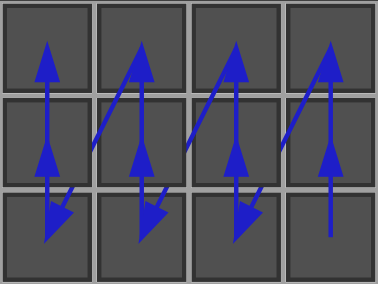Sequence of visited pixels in a scan of Khalimsky Space (other scan order).
• Translating arbitrarily a cell in the space with KhalimskySpaceND::uTranslation and KhalimskySpaceND::sTranslation.

# Cell topology and directions

As said above, the cell topology is defined by the parity of its Khalimsky coordinates. The number of coordinates where the cell is open define the dimension of the cell. A cell of maximal dimension (n) is called a pixel in 2D, a voxel in 3D, and sometimes called a spel or xel in nD. A cell of minimial dimension (0) is often called a pointel. n-1 cells are called surfels (or sometimes linels in 2D). Here are the methods related to the cell topology.

...
for ( KSpace::DirIterator q = ks.uDirs( p ); q != 0; ++q )
{
Dimension dir = *q;
...
}
typename PreCellularGridSpace::DirIterator DirIterator
DGtal::uint32_t Dimension
Definition: Common.h:136

You may obtain the cells of same topology which are (face-)adjacent to a given cell with the following methods:

# Cell incidence

Cells incident to some cell touch the cell but do not have the same dimension. Cells lower incident to a cell have lower dimensions, cells upper incident to a cell have higher dimensions. Specific rules determine the sign of incident cells to a signed cell:

1. A first rule is that along some axis, the forward and the backward incident cell have opposite sign.
2. A second rule is that the rules are invariant by translation of cells.
3. A third rule is that changing the sign of the cell changes the sign of all incident cells.
4. A last rule is that taking an incident cell along some axis k then taking an incident cell along some other axis l will give a cell that has the opposite sign as if the incidence was taken before along l and after along k.

These rules together allow the definition of (co)boundary operators, which are homomorphisms between chains of cells. They have the property that applied twice they give the null chain. Otherwise said, the boundary of a set of cells has an empty boundary.

SCell pix = K.sSpel( Point( 0,0 ), K.POS ); // pixel (0+1,0+1)
SCell leftl = K.sIncident( pix, 0, false ); // linel (0,0+1)
SCell rightl = K.sIncident( pix, 0, true ); // linel (2,0+1)
SCell downl = K.sIncident( pix, 1, false ); // linel (0+1,0)
SCell upl = K.sIncident( pix, 1, true ); // linel (0+1,2)
SCell ptld = K.sIncident( leftl, 1, false );// pointel (0,0)
SCell ptdl = K.sIncident( downl, 0, false );// pointel (0,0)
// ptld and ptdl have opposite signs.
Z3i::SCell SCell
// c is a signed cell, k a direction
ASSERT( K.sSign( K.sIncident( c, k, K.sDirect( c, k ) ) ) == K.POS );

# Periodic Khalimsky space and per-dimension closure specification.

In addition to the CCellularGridSpaceND requirements, KhalimskySpaceND allows the use of different closures for each dimension and the use of periodic dimension.

The closure is specified at the space initialization:

K.init( {-3, -2}, {2, 4}, { K.CLOSED, K.PERIODIC } ); // K is closed along the first dimension, but periodic along the second.
Aim: This class is a model of CCellularGridSpaceND. It represents the cubical grid as a cell complex,...

Therefore, any coordinates are valid along a periodic dimension but the coordinates accessors of KhalimskySpaceND will always return a coordinate between the two bounds given at the initialization:

KSpace::Cell cell = K.uSpel( {0, 6} ); // Valid, thanks to the periodicity.
int y = K.uCoord( cell, 1 ); // y = -1
auto cell2 = K.uGetSub( cell, 1, 2 ); // Decrementing second digital coordinate by 2.
int y2 = K.uCoord( cell2, 1 ); // y2 = 4

It is also possible to span a sub-space that crosses the bounds along a periodic dimension:

KSpace::Cell first = K.uSpel( {-2, 1} );
KSpace::Cell last = K.uSpel( { 2, 7} );
auto p = first;
do
{
// do something with the current cell [p]
}
while ( K.uNext( p, first, last ) );

# Unbounded cellular grid space

If you need to arbitrarily manipulate the cells coordinates without bounds checking, or if you simply doesn't need a bounded space, you may consider KhalimskyPreSpaceND.

It is a model of CPreCellularGridSpaceND, that is a base concept of CCellularGridSpaceND. The main difference is that CPreCellularGridSpaceND doesn't require to initialize the space with bounds and some bounds related methods (like KhalimskySpaceND::uGetMin) are not available.

Therefore, KhalimskyPreSpaceND is a purely static class that provides almost all the methods of KhalimskySpaceND but without bounds influence (coordinates validity, restricted neighborhood, ...) and is also fully compatible with KhalimskySpaceND-based algorithms when bounds are not necessary.

The main features are: